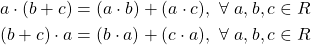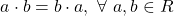# Mathematics - Discrete Mathematics - Rings

in STEMGeekslast year (edited)[Image 1]

## Introduction

Hey it's a me again @drifter1!

Today we continue with Mathematics, and more specifically the branch of "Discrete Mathematics", in order to get into Rings.

So, without further ado, let's get straight into it!

## Rings

Rings are yet another algebraic structure, which is defined as a set together with two binary operations on that set. The binary operations have to satisfy properties similar to those of addition and multiplication of integers. It's thus common to note these binary operations as "+" and "." respectively. Rings are thus denoted as (R, +, .), where R is the set and "+", "." the binary operations on that set.

### Ring Axioms

Rings satisfy the following three axioms:

• (R, +) is an abelian group, which means that it satisfies:
• associativity
• commutativity
• (R, .) is an monoid, which means that it satisfies:
• associativity
• (multiplicative) identity
• Multiplication (.) is distributive over addition (+)

Distributivity is the following property:### Commutative Rings

Commutative rings are rings, which additionally satisfy commutativity under the operation of multiplication, basically:### Non-commutative Rings

Rings which don't satisfy commutativity under either of the two binary operations are known as non-commutative rings.

For example, in the case of any ring R and natural number n, the set of all square n x n matrices forms a ring under matrix addition and multiplication, which is non-commutative.

### Semirings

Rings which don't satisfy additive invertibility are known as Semirings.

For example, the set of natural numbers together with addition and multiplication is a semiring, because not all elements are invertible under addition.

### Subrings

A subset A of a ring (R, +, .) is a subring of R if (A, +) is a subgroup of (R, +) and A is closed under multiplication.

In that context, a set consisting only of 0 or {0} and of course R itself are directly also subrings of R.

### Rng

In some definitions rings are defined without the requirement of multiplicative identity. Such structures are more commonly known as rng's.

## RESOURCES:

### Images

Block diagrams and other visualizations were made using draw.io.

## Previous articles of the series

• Introduction → Discrete Mathematics, Why Discrete Math, Series Outline
• Sets → Set Theory, Sets (Representation, Common Notations, Cardinality, Types)
• Set Operations → Venn Diagrams, Set Operations, Properties and Laws
• Sets and Relations → Cartesian Product of Sets, Relation and Function Terminology (Domain, Co-Domain and Range, Types and Properties)
• Relation Closures → Relation Closures (Reflexive, Symmetric, Transitive), Full-On Example
• Equivalence Relations → Equivalence Relations (Properties, Equivalent Elements, Equivalence Classes, Partitions)
• Partial Order Relations and Sets → Partial Order Relations, POSET (Elements, Max-Min, Upper-Lower Bounds), Hasse Diagrams, Total Order Relations, Lattices
• Combinatorial Principles → Combinatorics, Basic Counting Principles (Additive, Multiplicative), Inclusion-Exclusion Principle (PIE)
• Combinations and Permutations → Factorial, Binomial Coefficient, Combination and Permutation (with / out repetition)
• Combinatorics Topics → Pigeonhole Principle, Pascal's Triangle and Binomial Theorem, Counting Derangements
• Propositions and Connectives → Propositional Logic, Propositions, Connectives (∧, ∨, →, ↔ and ¬)
• Implication and Equivalence Statements → Truth Tables, Implication, Equivalence, Propositional Algebra
• Proof Strategies (part 1) → Proofs, Direct Proof, Proof by Contrapositive, Proof by Contradiction
• Proof Strategies (part 2) → Proof by Cases, Proof by Counter-Example, Mathematical Induction
• Sequences and Recurrence Relations → Sequences (Terms, Definition, Arithmetic, Geometric), Recurrence Relations
• Probability → Probability Theory, Probability, Theorems, Example
• Conditional Probability → Conditional Probability, Law of Total Probability, Bayes' Theorem, Full-On Example
• Graphs → Graph Theory, Graphs (Vertices, Types, Handshake Lemma)
• Graphs 2 → Graph Representation (Adjacency Matrix and Lists), Graph Types and Properties (Isomorphic, Subgraphs, Bipartite, Regular, Planar)
• Paths and Circuits → Paths, Circuits, Euler, Hamilton
• Trees → Trees (Rooted, General and Binary), Tree Traversal, Spanning Trees
• Common Graph Problems → Shortest Path Problem, Graph Connectivity, Travelling Salesman Problem, Minimum Spanning Tree, Maximum Network Flow, Graph Coloring
• Binary Operations → Binary Operations (n-ary, Table Representation), Properties
• Groups → Groups (Properties, Theorems, Finite and Infinite, Abelian, Cyclic, Product, Homo-, Iso- and Auto-morphism)
• Group-like Structures (part 1) → Subgroups, Semigroups, Monoids
• Group-like Structures (part 2) → Magma, Quasigroup, Groupoid

## Final words | Next up

And this is actually it for today's post!

Next time we will cover Fields...

See ya!Keep on drifting!

Posted with STEMGeeks

Sort:

As always, great explanation!

!1UP#### You have received a 1UP from @luizeba!

The following @oneup-cartel family members will soon upvote your post:
`@stem-curator`
And they will bring !PIZZA 🍕